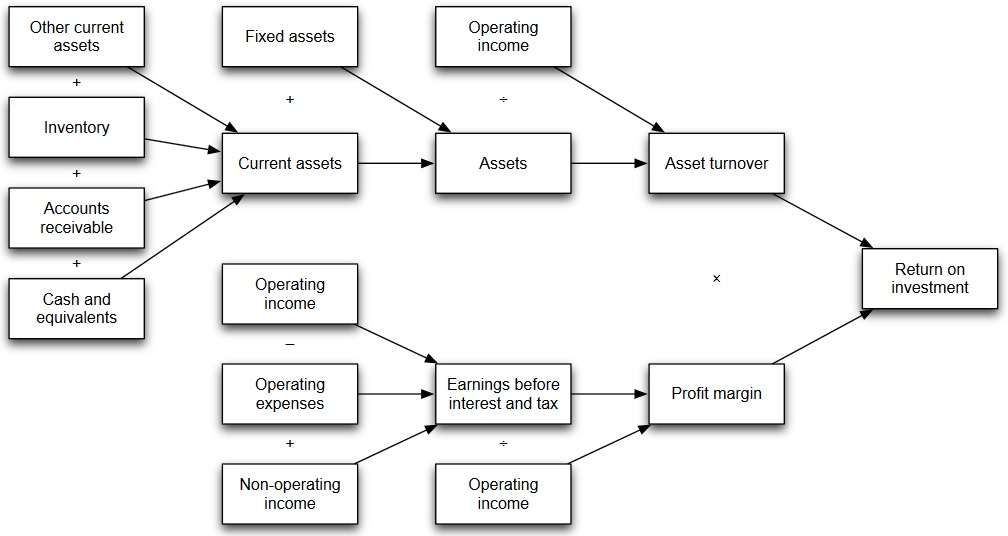# DuPont Finance Analysis

This way to perform financial analysis started to be done by DuPont in 1920, and it gained a lot of recognition and acceptance.

• Profitability (measured by profit margin) = Net profit/Sales
• Operating efficiency (measured by asset turnover) = Sales/Assets
• Financial leverage (measured by equity multiplier) = Assets/Equity

The calculation sequence is as follows:

• ROE = (Profit margin) * (Asset turnover) * (Equity multiplier)
• ROE = (Net profit/Sales)*(Sales/Assets)*(Assets/Equity)
• ROE = (Net Profit/Equity)

The breakdown is shown below: